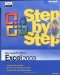# Key Points

• You can add a group of cells to a formula by typing the formula and then, at the spot in the formula where you want to name the cells, selecting the cells using the mouse.

• Creating named ranges lets you refer to entire blocks of cells with a single term , saving you lots of time and effort.

• When you write a formula, be sure you use absolute referencing (\$A\$1) if you want the formula to remain the same when its copied from one cell to another, or relative referencing (A1) if you want the formula to change to reflect its new position in the worksheet.

• Rather than type in a formula from scratch, you can use the Insert Function dialog box to help you on your way.

• You can monitor how the value in a cell changes by adding a watch to the watch window.

• To see which formulas refer to the values in the selected cell, use Trace Dependents; if you want to see which cells provide values for the formula in the active cell, use Trace Precedents.

• You can step through the calculations of a formula in the Evaluate Formula dialog box, or go through a more rigorous error-checking procedure using the Error Checking tool.MicrosoftВ® Office ExcelВ® 2003 Step by Step (Step By Step (Microsoft))
ISBN: 0735615187
EAN: 2147483647
Year: 2005
Pages: 350
Authors: Curtis Frye

Similar book on Amazon